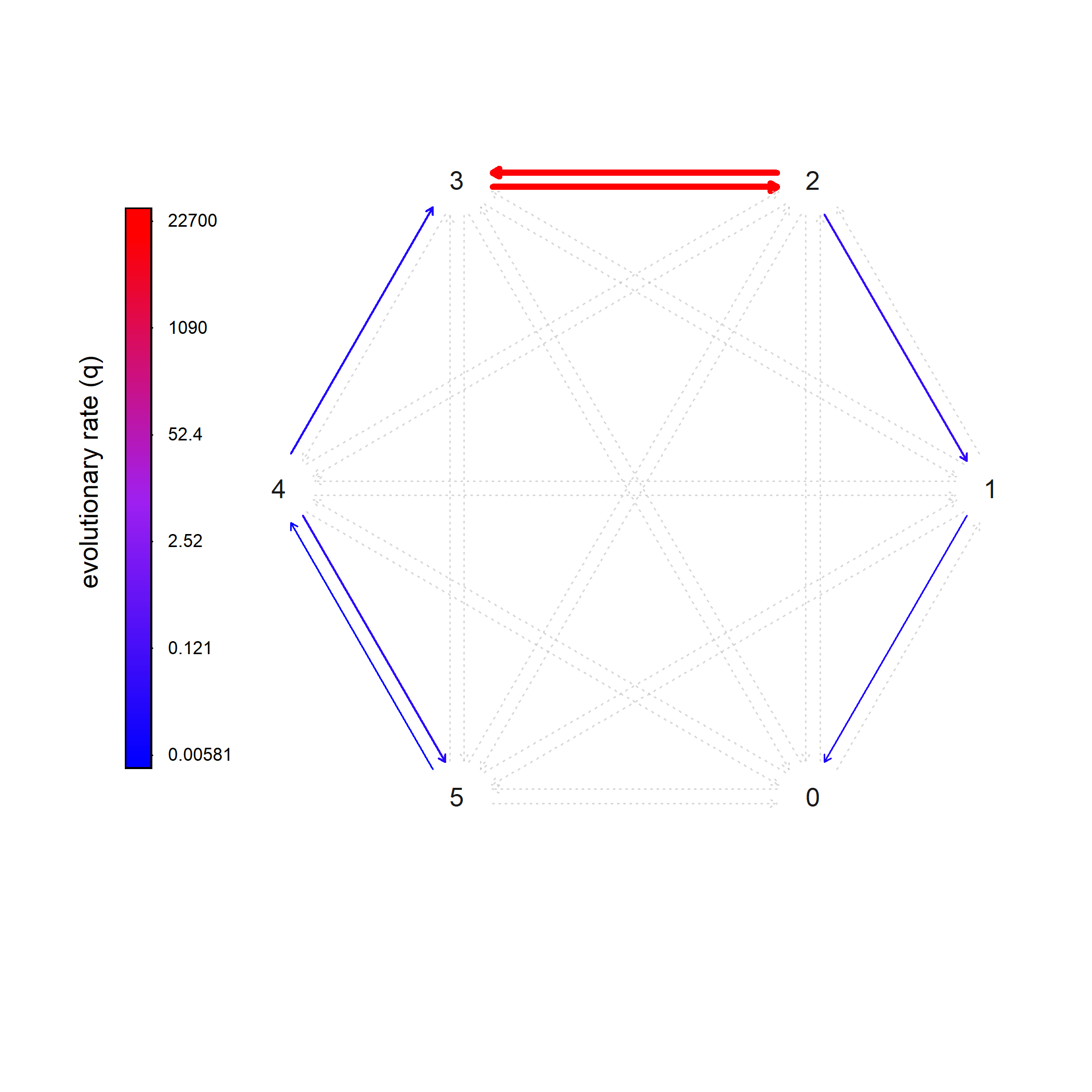## Sunday, April 16, 2023

### Fitting an ordered discrete character evolution model using `fitHRM`

The phytools function `fitHRM` is designed primarily to fit various flavors of the hidden-rates model of Beaulieau et al. (2013).

It also, however, turns out to be a fairly robust & convenient way to fit an ordered Mk model because one of the classes of model (what I have called the umbral model, but is actually a generalization of Marazzi et al.‘s 2012 precursor model) has an “ordered” version, and if we set the number of rate classes to 1, we’re just fitting a standard ordered Mk model without hidden rates!

Here’s a simple example using the number of the digits in the hindfeet of squamate reptiles, adapted from Brandley et al. (2008).

``````library(phytools)
library(geiger)
row.names=1)
chk<-name.check(squamate.tree,squamate.data)
squamate.tree<-drop.tip(squamate.tree,chk\$tree_not_data)
rear_toes<-setNames(squamate.data[squamate.tree\$tip.label,],
squamate.tree\$tip.label)
``````
``````ordered_Mk<-fitHRM(squamate.tree,rear_toes,niter=10,
parallel=TRUE,ncores=10,umbral=TRUE,ordered=TRUE,
order=sort(unique(rear_toes)),pi="fitzjohn",ncat=1,
logscale=TRUE)
``````
``````##
## This is the design matrix of the fitted model.
## Does it make sense?
##
##   0 1 2 3  4 5
## 0 0 1 0 0  0 0
## 1 2 0 3 0  0 0
## 2 0 4 0 5  0 0
## 3 0 0 6 0  7 0
## 4 0 0 0 8  0 9
## 5 0 0 0 0 10 0
##
## Opened cluster with 10 cores.
## Running optimization iterations in parallel.
``````
``````ordered_Mk
``````
``````## Object of class "fitHRM".
##
## Observed states: [ 0, 1, 2, 3, 4, 5 ]
## Number of rate categories per state: [ 1, 1, 1, 1, 1, 1 ]
##
## Fitted (or set) value of Q:
##          0         1         2             3         4         5
## 0 0.000000  0.000000      0.00      0.000000  0.000000  0.000000
## 1 0.022288 -0.022288      0.00      0.000000  0.000000  0.000000
## 2 0.000000  0.066147 -18160.30  18160.233185  0.000000  0.000000
## 3 0.000000  0.000000  22700.37 -22700.366700  0.000000  0.000000
## 4 0.000000  0.000000      0.00      0.026384 -0.066295  0.039910
## 5 0.000000  0.000000      0.00      0.000000  0.005813 -0.005813
##
## Fitted (or set) value of pi:
##        0        1        2        3        4        5
## 0.000000 0.000000 0.000000 0.000000 0.275171 0.724829
## due to treating the root prior as (a) nuisance.
##
## Log-likelihood: -114.967167
##
## Optimization method used was "nlminb"
``````

That’s all there is to it.

Something weird happens if we call `plot` on this object, though, because it has the class attributes `fitHRM` and `fitMk`.

Let’s see.

``````plot(ordered_Mk,asp=0.5,offset=0.03)
``````To get our more classic looking Mk plot, all we need to do is convert our fitted model object to an object of class `"Qmatrix"` and then plot that!

``````plot(as.Qmatrix(ordered_Mk),width=TRUE,color=TRUE,xlim=c(-1.5,1),
text=FALSE,max.lwd=4)
``````Cool. Alright.